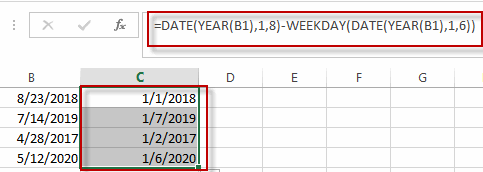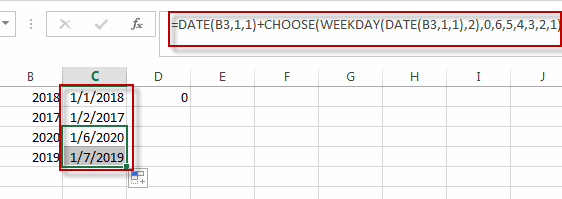# Get the First Monday of a Given Year

This post will guide you how to get the first Monday of a given year or date in excel. How do I calculate the first Monday of a specific year in excel. How to determine the first Monday in a given year date with a formula in excel. How to find and get the date of the first Monday of a given year date in excel.

## Get the First Monday of a Given Year

To calculate the first Monday of the year or given any date, you can create a new complex formula based on the DATE function, the YEAR function and the WEEKDAY function.

Assuming that you have a date list in the range of cells B1:B4 in your current worksheet, and if you want to get the first Monday for the year date in each cell, and you can write down one formula as below:

`=DATE(YEAR(B1),1,8)-WEEKDAY(DATE(YEAR(B1),1,6))`Type this formula into the formula box of a blank cell such as: C1, then press Enter key in your keyboard, and drag the AutoFill Handler over other cells to apply this formula.

You can also use another formula based on the DATE function, the CHOOSE function and the WEEKDAY function to create the same result. Like this:

`=DATE(B1,1,1)+CHOOSE(WEEKDAY(DATE(B1,1,1),2),0,6,5,4,3,2,1)`### Related Functions

• Excel WEEKDAY function
The Excel WEEKDAY function returns a integer value representing the day fo the week for a given Excel date and the value is range from 1 to 7.The syntax of the WEEKDAY function is as below:=WEEKDAY (serial_number,[return_type])…
• Excel DATE function
The Excel DATE function returns the serial number for a date.The syntax of the DATE function is as below:= DATE (year, month, day)…
• Excel YEAR function
The Excel YEAR function returns a four-digit year from a given date value, the year is returned as an integer ranging from 1900 to 9999. The syntax of the YEAR function is as below:=YEAR (serial_number)…
• Excel Choose Function
The Excel CHOOSE function returns a value from a list of values. The CHOOSE function is a build-in function in Microsoft Excel and it is categorized as a Lookup and Reference Function.The syntax of the CHOOSE function is as below:=CHOOSE (index_num, value1,[value2],…)…
Related Posts

Calculating Average Of The Numbers

Are you weary of investing a lot of time and effort in manually calculating the average of the numbers by including or excluding 0 and calculating the average of the top 3 scores? Then congratulations because you have just landed ...

Add Months To Date In Excel

It is important to adjust time periods when performing financial modeling. In Excel, you can use the MONTH function to add a specific number of months to a date. The MONTH function takes one argument: the number of months you ...

Add Days to Date in MS Excel

If you are an vavid MS Excel user, then you might have come across situations where you need to add the same or different days into the particular date, and if you have done this task manually, then let me ...

Filter Data By Date Field

The use of Microsoft Excel's Format Cells and Auto Filter option is a great way to filter data by year or Month. This article will discuss how to filter by date field using Filter function and Format cells in Microsoft ...

How to Count Dates of Given Year in Excel

This post will guide you how to count Dates of a certain year in the range of dates using a formula in Excel 2013/2016 or Excel office 365. How do I count dates by a given year in Excel. And ...

How to Sum Values Based on Month and Year in Excel

We often do some summary or statistic at the end of one month or one year. In these summary tables, there are at least two columns, one column records the date, and the other column records the sales or product ...

How to Convert Date & Time Format to Date in Excel

Sometimes we want to convert date and time format to date only format in excel for example convert 01/29/2019 06:51:03 to 01/29/2019, we can convert format by Formula or Format Settings. The two ways are easy to learn, so you ...

How to Extract Year from Date & Time Format in Excel

Sometimes we want to get Year information from date and time format to show the Year only in excel. For example convert 01/29/2019 06:51:03 to 2019, we can get Year information by Formula or Format Settings. The two ways are ...

How to Calculate Remaining Days in a Month or Year in Excel

This post will guide you how to calculate remaining days in a given month or year in Excel. How do I calculate the number of days left in a month or year using a formula in Excel 2013/2016. Calculate Remaining ...

How to Split Date into Day, Month and Year in Excel

This post will guide you how to split date into separate day, month and year in excel. How do I quickly split date as Day, Month and Year using Formulas or Text to Columns feature in Excel. Split Date into ...

Sidebar